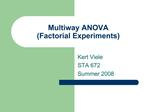# Multiway ANOVA Factorial Experiments - PowerPoint PPT PresentationDownload PresentationMultiway ANOVA Factorial Experiments

Multiway ANOVA Factorial ExperimentsDownload Presentation## Multiway ANOVA Factorial Experiments

- - - - - - - - - - - - - - - - - - - - - - - - - - - E N D - - - - - - - - - - - - - - - - - - - - - - - - - - -
##### Presentation Transcript

1. Multiway ANOVA (Factorial Experiments) Kert Viele STA 672 Summer 2008

2. Multiway ANOVA It is certainly possible to consider more than one categorical variable at once. In fact, if you are interested in the effect of several variables, you can get much more information out of a carefully designed experiment manipulating all the variables than separate experiments manipulating each variable separately.

3. Two or more categorical variables In STA671, we considered two categorical variables at a time, often with some continuous variables mixed in for fun (ANCOVA). In STA672, we will discuss this in more generality, allowing several categorical variables at once. Many of the principles (residual plots, backward elimination, etc.) remain the same, just more complicated to implement.

4. Some things never change One rule that remains constant is that using multiple factors, you still cannot fit more than one continuous variable in an interaction (that would have a different interpretation). Any number of categorical variables is allowed. Another rule that remains constant is that you cannot remove protected terms. If a term is contained inside another term, it is protected.

5. More things that don�t change Aside from those rules, a backward method of selection is just as before. Find all terms that are neither protected nor significant. If there are any, remove the one with the highest p-value. If there are not (all unprotected terms are significant), then you have your final model. Always check your residual plot! The same principles continue to apply (look for outliers, curvature, and changing variance)

6. Twoway ANOVA Suppose we can classify observations into groups on the basis of two variables, such as gender and race. Let Yijk be the kth observation in the group with first variable equal to i and second variable equal to j. There are potentially effects due to both variables.

7. Twoway ANOVA One way to incorporate both variables is the twoway ANOVA with Yijk = � + ai + �j + eijk The mean of group ij is �ij = � + ai + �j As with oneway ANOVA, there is a �base case� parameterization and an �ANOVA� parameterization.

8. Base case parameterization Suppose you had the table below. In the �base case� parameterization, you would pick a row and column (arbitrary decision, doesn�t much which) to use as the �base group�. Let�s arbitrarily pick the second row and the third column as the base groups. This means we set a2=0 and �3=0. This forces �=48, a1=(-18), a2=0, �1=(-15), �2=(-3), and �3=0. The parameter �=48 simply refers to the base group, and the other parameters are adjustments.

9. ANOVA parameterization Suppose you had the table below. In the �ANOVA� parameterization, the parameter � would be the average of all the groups, here �=33. This forces �=33, a1=(-9), a2=9, �1=(-9), �2=3, �3=6. Remember, it�s all ways of expressing the 6 numbers in the table, you get to the same place. I will tend to use the base case formulation. I think it�s simpler and what SAS uses.

10. Interaction, ugh, yuck, NOOOO! Unfortunately, the model Yijk = � + ai + �j + eijk is often not general enough. Consider a simple example where I=2 and J=3. A table with the mean values below �=33, a1=(-9), a2=9, �1=(-9), �2=3, �3=6

11. Additive models come with restrictions Note in the table below (same as last slide) the differences between the rows are the same in each column (so 33-15=18, 45-27=18, and 48-30=18) This isn�t by accident, it ALWAYS happens when using the additive model

12. Interaction Unfortunately, the table below is not recoverable. If the difference between the rows differs depending on which column you are in (33-15=18, 45-27=18, but 54-30=24), this is called an �interaction�. The solution is to include more parameters to make up the difference

13. Interactions Yijk = � + ai + �j + ?ij + eijk The ? terms measure the difference between the additive model and the actual means. To achieve complete generality, we don�t need a ? for every row/column combination, just the ones that do not correspond to the baseline cases. Great, so what do they mean?

14. Meaning of Interaction terms When interaction terms are included, this indicates that the difference between the rows changes depending on the column. Suppose we had 2 variables, age and gender, and we observe people with age=12 and age=30. If the response variable is height, an interaction is present. The difference between men and women�s height is different at age=12 than at age=30.

15. Fitted values for Yijk = � + ai + �j + ?ij + eijk with I=1, J=1 as baseline. Every cell can be fit with it�s own mean.